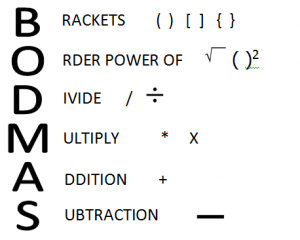# BODMAS rule also known as BIDMASBODMAS is an backronym which helps in remembering the order of operations in mathematical calculations. This is the order in which you should simplify the expression.  It stands for 'Brackets, Order, Division, Multiplication, Addition, Subtraction.'  When you have a complex problem to simplify, you need to use this principle.

BODMAS rule of solving:

1. First you begin by simplifying the expressions within the brackets.  Note BODMAS needs to be applied to the expression within the bracket as well.
2. Simplify the power of indices.
3. Division
4. Multiplication